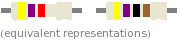# WolframAlpha guide on electrical engineering

waht
Introduction:

WolframAlpha is a freely available computational engine that can supplement already existing software such as mathematica, maple, mathlab, or excel. The purpose of this mini-guide is to go over some of its functionality with emphasis on electrical engineering.

www.wolframalpha.com

Dimensional analysis:

WolframAlpha recognizes many common units. It will simplify dimensions and scale units. For example,

Code:
farad * henry
will generate [time]^2

Code:
0.01 uF to pF
will generate 10,000 pF or 10 nF

WolframAlpha also can tap its database to pull up a conversion formula from the context

Code:
convert 25 MHz to meters
will convert the frequency to wavelength 12 meters

Code:
find energy in 100 uF at 10 KV
will give energy in a capacitor,

or to find inductance to resonate with 10 nF capacitor at 100 Khz; just say that

Code:
find inductance for 10 nF at 100 KHz
output 253 nH

Also if you haven't memorized resistor color bands already, you can still look them up

Code:
resistor yellow purple red

output: 4.7K

Complex numbers and phasors:

Working in polar form is supported in W|A. By typing an impedance in rectangular form

Code:
30 + 45i
we are quickly given its magnitude and phase of 54, and 56 degrees

and likewise, we can indicate a phasor in polar form with an exponential e

Code:
e^(i pi)
generates -1

we can also manipulate phasors algebraically

Code:
100 e^(i 45 deg) + 25 e^(i 30 deg)
output: magnitude = 124, phase = 42 deg

indicate degrees with “deg” otherwise W|A will interpret it in radians.

Solving equations:

To solve $x^2 - 2x + 1= 0$, enter the equation as it is

Code:
x^2 - 2x + 1 = 0
output x = 1

and W|A solves it. Don't even have to specify the variable to be solved.

Solving systems of equations is just as easy,

$$v_1 + v_2 + v_3 = 4$$

$$v_1 - v_2 = 10$$

$$v_1 - 3v_2 + 5v_3 = 8$$

simply type

Code:
v1 + v2 + v3 = 4, v1-v2 = 10, v1 - 3v2 + 5v3 = 8
output v1 = 23/3, v2 = -7/3, v3 = -4/3

just make sure that the equations are separated by a comma.

Differential equations are supported as well. An nth number of apostrophes indicates an nth derivative, and proceed as before. For example to solve

$$\frac{d^2y}{dx^2} - \frac{dy}{dx} - 2y = 0 \indent y(0) = 1, y'(0) = 2$$

enter the code as follows,

Code:
y'' – y' - 2 y = 0, y(0) = 1, y'(0) = 2
and we get a solution y = e^2x as well as a plot that can be saved in pdf format, and printed out.

We can also maximize and minimize

Code:
max 1 - x^2
finds maximum of 1

Logic gates and boolean algebra:

Converts number bases,

Code:
binary 1111101 to hex
= 7d

We can also work with boolean expression in WolramAlpha, for example

Code:
(x or y) and (x or !y)
generates a truth table:

http://img200.imageshack.us/img200/9384/truthtable.gif [Broken]

a schematic with logic gates, and even simplifies the expression to a minimal form

http://img268.imageshack.us/img268/3233/scehmatic.gif [Broken]

Here is the supported syntax:
Code:
NOT = !
OR = ||
AND = &&

NAND
NOR
XOR

More on calculus:

W|A can perform basic calculus

take derivatives:

$$\frac{d}{dt} cos(t)$$

Code:
derivative cos(t)

do integration:

$$\int \frac{1}{x+1} dx$$

Code:
integrate 1/(x+1) dx

and take Laplace and Fourier transforms:

$$\mathcal{L}(x^2), \mathcal{F}(e^x)$$

Code:
laplace x^2
Code:
fourier  e^x

Plotting:

If you want to graph a transfer function for instance,

$$H(s) = \frac{s+10}{s^2 + 4s + 8}$$

enter:

Code:
 graph (s + 10)/(s^2 + 4s + 8)

and here we have

http://img32.imageshack.us/img32/9715/graph.gif [Broken]

Conclusion:

WolframAlpha uniquely combines different tools into a one freely available package and that expands our tool box. This mini-guide doesn't cover all of W|A, it merely touches upon features that can be used in electrical engineering, for more information visit their examples page

http://www28.wolframalpha.com/examples/

also, W|A is said to expand in the future, enabling more functionality, and tools.

Last edited by a moderator:

Mentor
Thanks for putting that together, waht. I've stickied this thread to help us learn how to utilize W|A.

Any further posts in this thread should be about tips on how to use W|A and its features. Discussions about W|A and its implications for eductaion, etc., belong in a different thread, like this one: https://www.physicsforums.com/showthread.php?t=307686

.

Last edited:
Staff Emeritus
Homework Helper
Resistor color codes

Also if you haven't memorized resistor color bands already, you can still look them up

Code:
resistor yellow purple red

output: 4.7K

The inverse operation works also. Type this for input:
Code:
resistor color 4.7k ohms
... and the output is an image of the resistor with the correct color bands:View the full W|A output here:
http://www5f.wolframalpha.com/input/?i=resistor+color+4.7k+ohms&asynchronous=false&equal=Submit [Broken]
.
.

#### Attachments

Last edited by a moderator:
Gold Member
Check out the WolframAlpha app for the iPhone and iPod Touch. Its pretty awesome!

rathat
Remember when the WA app cost $50? They thought because it's better than any scientific calculator could be, they could charge that much, but you know, no one would pay that much so they made it$2.

Ecin
is there any advantage over the ipod app vs the standard web version?

CenterFusion
Would you guys recommend wolfram alpha to a student who is interested in learning more about physics and modeling physics within computing systems? I have a small bit of experience with mathlab, and am hoping to be on the go as I do this (iPad, Android, etc.)

m.s.j
I have "Algeo.apk" in my galaxy tab, it is a wonderful application in small scale.